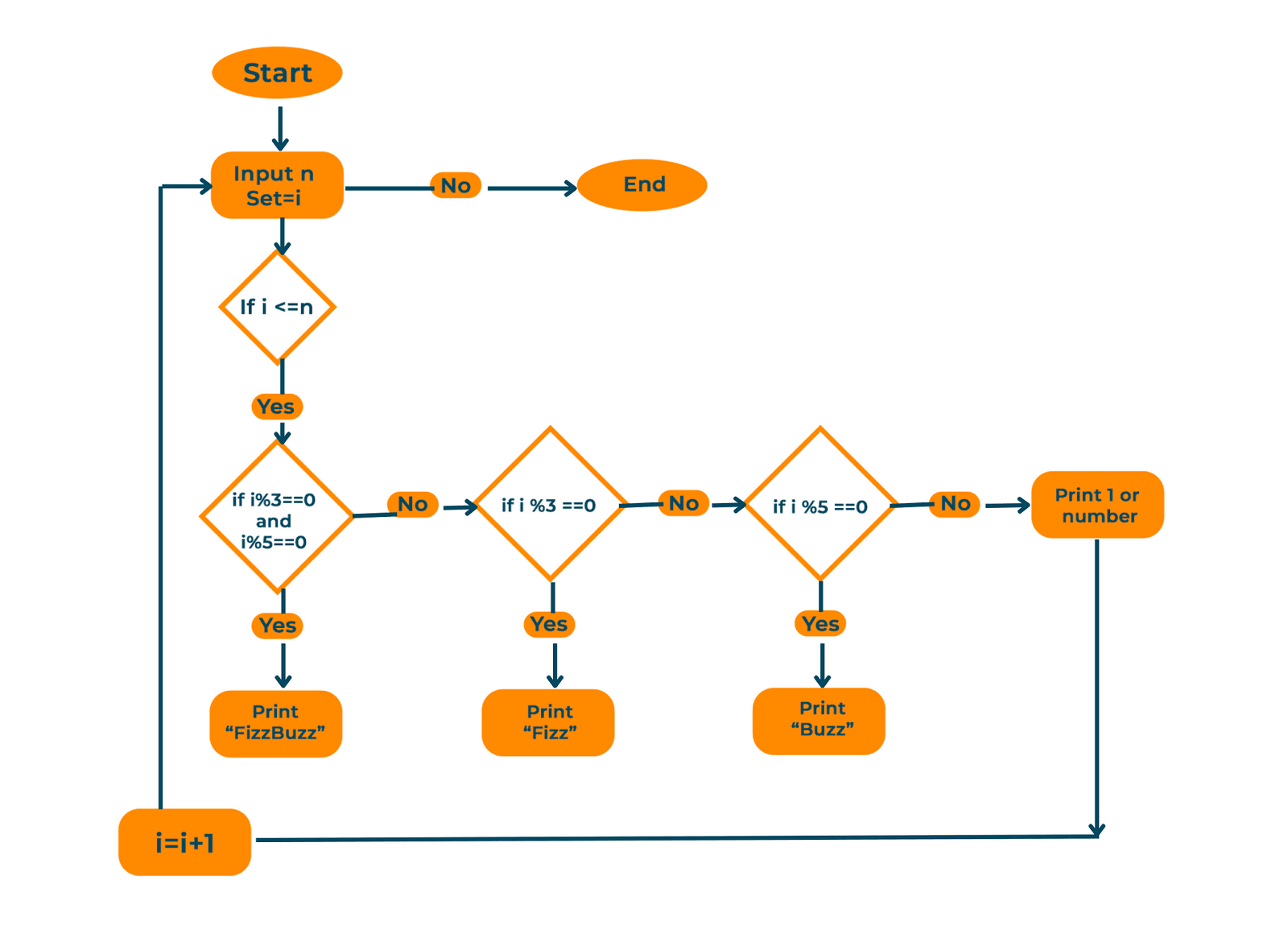# FizzBuzz Problem & Solution in Python (& Flowchart)

FizzBuzz is a well-known coding problem that frequently appears in entry-level job interviews. We'll go into the specifics of the FizzBuzz problem in this article and explain how to write FizzBuzz code in Python.

## FizzBuzz Problem Statement

FizzBuzz problems test your logical thinking and how well you know loops and control flow. Here is the problem statement:

"Write a program that prints the numbers from 1 to n. But for multiples of 3, print 'Fizz' instead of the number, and for multiples of 5, print 'Buzz'. For numbers that are multiples of both 3 and 5, print 'FizzBuzz'."

Sample Output:

```1
2
Fizz
4
Buzz
Fizz
7
8
Fizz
Buzz
11
...
...
...
```

### Flowchart & Pseudocode

Here is the full flowchart for the FizzBuzz problem to easily understand it.The pseudocode for the above problem statement:

1. Start
2. Take ‘n’ as input from the user
3. Initialize i as 1
4. Run a loop i till N+1:
5. If i % 5 == 0 and i % 3 == 0 then print “FizzBuzz”
6. Elif i % 3 == 0 then print “Fizz”
7. Elif i % 5 ==0 then print “Buzz”
8. Else print i
9. End

### FizzBuzz Python Solution

Here is the Python code to solve the FizzBuzz problem:

```# Fizzbuzz Problem
n=int(input("Enter the last range of numbers for FizzBuzz Problem :"))
for i in range(1,n+1):
if i % 3 == 0 and i % 5 == 0:
print("FizzBuzz")
elif i % 3 == 0:
print("Fizz")
elif i % 5 == 0:
print("Buzz")
else:
print(i)
```

Output:

For input n=50

```1
2
Fizz
4
Buzz
Fizz
7
8
Fizz
Buzz
11
Fizz
13
14
FizzBuzz
16
17
Fizz
19
Buzz
Fizz
22
23
Fizz
Buzz
...
47
Fizz
49
Buzz
```

We start by using a for loop to iterate over the numbers from 1 to n, where n is imputed by the user for each number, we use the modulus operator (%) to check if it's a multiple of 3, 5, or both by comparing its remainder value with 0.

If the number is a multiple of both 3 and 5, we print "FizzBuzz". If it's only a multiple of 3, we print "Fizz". If it's only a multiple of 5, we print "Buzz". If it's not a multiple of either, we just print the number.

This is the best method for this problem but what if the interviewer asked you to solve it without using any built-in operator like modulo (%),

You can solve this problem by maintaining a counter variable for every iteration your number passes. Here is an example:

```n=int(input("Enter the last range of numbers for FizzBuzz Problem :"))
count3=1
count5 = 1
for i in range(1,n+1):
if count3 == 3 and count5 == 5:
print("FizzBuzz")
count3,count5 = 0,0
elif count3 == 3:
print("Fizz")
count3 = 0
elif count5 == 5:
print("Buzz")
count5= 0
else:
print(i)
count3 += 1
count5 += 1
```

Output:

For input n=15

```1
2
Fizz
4
Buzz
Fizz
7
8
Fizz
Buzz
11
Fizz
13
14
FizzBuzz
```

Here, The user is prompted to enter the last number in the range of numbers that will be tested for the FizzBuzz issue by the code at the outset. Then, it sets the starting value of count3 and count5 to 1. The code then uses a for loop to cycle through the integers from 1 to the range that the user provided (n). The code determines whether count3 and count5 are equal for each number.

• The code writes "FizzBuzz" and sets the counts to 0 if both requirements are met.
• The code writes "Fizz" and sets count3 to 0 if count3 equals 3 but count5 does not equal 5.
• The code writes "Buzz" and sets count5 to 0 if count5 equals 5 but count3 does not.
• The code just prints the current number in the event that count3 or count5 is not equal to their corresponding multiples.

Lastly, the code raises count3 and count5 by 1 with each loop iteration. This method checks for multiples of 3 and 5, just like the modulo method does, but instead of using the modulo operator, it utilizes two counts that are increased with each loop iteration.

## Conclusion

The FizzBuzz issue is a straightforward but efficient approach to gauging a programmer's proficiency with fundamental programming ideas. It is a wonderful problem to practice on if you're getting ready for a job interview. We learned everything about FizzBuzz in Python here! Happy Learning :)

### FavTutor - 24x7 Live Coding Help from Expert Tutors!